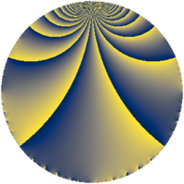# Properties

 Label 6720.2.a.cyLevel $6720$ Weight $2$ Character orbit 6720.a Self dual yes Analytic conductor $53.659$ Analytic rank $0$ Dimension $2$ CM no Inner twists $1$

# Related objects

## Newspace parameters

 Level: $$N$$ $$=$$ $$6720 = 2^{6} \cdot 3 \cdot 5 \cdot 7$$ Weight: $$k$$ $$=$$ $$2$$ Character orbit: $$[\chi]$$ $$=$$ 6720.a (trivial)

## Newform invariants

 Self dual: yes Analytic conductor: $$53.6594701583$$ Analytic rank: $$0$$ Dimension: $$2$$ Coefficient field: $$\Q(\sqrt{2})$$ Defining polynomial: $$x^{2} - 2$$ Coefficient ring: $$\Z[a_1, \ldots, a_{11}]$$ Coefficient ring index: $$2$$ Twist minimal: no (minimal twist has level 3360) Fricke sign: $$-1$$ Sato-Tate group: $\mathrm{SU}(2)$

## $q$-expansion

Coefficients of the $$q$$-expansion are expressed in terms of $$\beta = 2\sqrt{2}$$. We also show the integral $$q$$-expansion of the trace form.

 $$f(q)$$ $$=$$ $$q + q^{3} + q^{5} + q^{7} + q^{9} +O(q^{10})$$ $$q + q^{3} + q^{5} + q^{7} + q^{9} + \beta q^{11} + ( 2 + \beta ) q^{13} + q^{15} + ( 2 - 2 \beta ) q^{17} + \beta q^{19} + q^{21} + 4 q^{23} + q^{25} + q^{27} + ( 2 - 2 \beta ) q^{29} -\beta q^{31} + \beta q^{33} + q^{35} + ( 6 - 2 \beta ) q^{37} + ( 2 + \beta ) q^{39} + ( -2 + 2 \beta ) q^{41} + ( -4 - 2 \beta ) q^{43} + q^{45} + 4 \beta q^{47} + q^{49} + ( 2 - 2 \beta ) q^{51} + ( -2 + 3 \beta ) q^{53} + \beta q^{55} + \beta q^{57} + ( 2 + 4 \beta ) q^{61} + q^{63} + ( 2 + \beta ) q^{65} -4 q^{67} + 4 q^{69} + ( 8 - \beta ) q^{71} + ( -2 + \beta ) q^{73} + q^{75} + \beta q^{77} + ( 4 - 4 \beta ) q^{79} + q^{81} + ( -12 + 2 \beta ) q^{83} + ( 2 - 2 \beta ) q^{85} + ( 2 - 2 \beta ) q^{87} + ( 6 - 2 \beta ) q^{89} + ( 2 + \beta ) q^{91} -\beta q^{93} + \beta q^{95} + ( -10 - \beta ) q^{97} + \beta q^{99} +O(q^{100})$$ $$\operatorname{Tr}(f)(q)$$ $$=$$ $$2q + 2q^{3} + 2q^{5} + 2q^{7} + 2q^{9} + O(q^{10})$$ $$2q + 2q^{3} + 2q^{5} + 2q^{7} + 2q^{9} + 4q^{13} + 2q^{15} + 4q^{17} + 2q^{21} + 8q^{23} + 2q^{25} + 2q^{27} + 4q^{29} + 2q^{35} + 12q^{37} + 4q^{39} - 4q^{41} - 8q^{43} + 2q^{45} + 2q^{49} + 4q^{51} - 4q^{53} + 4q^{61} + 2q^{63} + 4q^{65} - 8q^{67} + 8q^{69} + 16q^{71} - 4q^{73} + 2q^{75} + 8q^{79} + 2q^{81} - 24q^{83} + 4q^{85} + 4q^{87} + 12q^{89} + 4q^{91} - 20q^{97} + O(q^{100})$$

## Embeddings

For each embedding $$\iota_m$$ of the coefficient field, the values $$\iota_m(a_n)$$ are shown below.

For more information on an embedded modular form you can click on its label.

Label $$\iota_m(\nu)$$ $$a_{2}$$ $$a_{3}$$ $$a_{4}$$ $$a_{5}$$ $$a_{6}$$ $$a_{7}$$ $$a_{8}$$ $$a_{9}$$ $$a_{10}$$
1.1
 −1.41421 1.41421
0 1.00000 0 1.00000 0 1.00000 0 1.00000 0
1.2 0 1.00000 0 1.00000 0 1.00000 0 1.00000 0
 $$n$$: e.g. 2-40 or 990-1000 Significant digits: Format: Complex embeddings Normalized embeddings Satake parameters Satake angles

## Atkin-Lehner signs

$$p$$ Sign
$$2$$ $$1$$
$$3$$ $$-1$$
$$5$$ $$-1$$
$$7$$ $$-1$$

## Inner twists

This newform does not admit any (nontrivial) inner twists.

## Twists

By twisting character orbit
Char Parity Ord Mult Type Twist Min Dim
1.a even 1 1 trivial 6720.2.a.cy 2
4.b odd 2 1 6720.2.a.cr 2
8.b even 2 1 3360.2.a.bc 2
8.d odd 2 1 3360.2.a.be yes 2

By twisted newform orbit
Twist Min Dim Char Parity Ord Mult Type
3360.2.a.bc 2 8.b even 2 1
3360.2.a.be yes 2 8.d odd 2 1
6720.2.a.cr 2 4.b odd 2 1
6720.2.a.cy 2 1.a even 1 1 trivial

## Hecke kernels

This newform subspace can be constructed as the intersection of the kernels of the following linear operators acting on $$S_{2}^{\mathrm{new}}(\Gamma_0(6720))$$:

 $$T_{11}^{2} - 8$$ $$T_{13}^{2} - 4 T_{13} - 4$$ $$T_{17}^{2} - 4 T_{17} - 28$$ $$T_{19}^{2} - 8$$ $$T_{23} - 4$$ $$T_{29}^{2} - 4 T_{29} - 28$$ $$T_{31}^{2} - 8$$

## Hecke characteristic polynomials

$p$ $F_p(T)$
$2$ $$T^{2}$$
$3$ $$( -1 + T )^{2}$$
$5$ $$( -1 + T )^{2}$$
$7$ $$( -1 + T )^{2}$$
$11$ $$-8 + T^{2}$$
$13$ $$-4 - 4 T + T^{2}$$
$17$ $$-28 - 4 T + T^{2}$$
$19$ $$-8 + T^{2}$$
$23$ $$( -4 + T )^{2}$$
$29$ $$-28 - 4 T + T^{2}$$
$31$ $$-8 + T^{2}$$
$37$ $$4 - 12 T + T^{2}$$
$41$ $$-28 + 4 T + T^{2}$$
$43$ $$-16 + 8 T + T^{2}$$
$47$ $$-128 + T^{2}$$
$53$ $$-68 + 4 T + T^{2}$$
$59$ $$T^{2}$$
$61$ $$-124 - 4 T + T^{2}$$
$67$ $$( 4 + T )^{2}$$
$71$ $$56 - 16 T + T^{2}$$
$73$ $$-4 + 4 T + T^{2}$$
$79$ $$-112 - 8 T + T^{2}$$
$83$ $$112 + 24 T + T^{2}$$
$89$ $$4 - 12 T + T^{2}$$
$97$ $$92 + 20 T + T^{2}$$